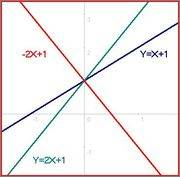# Meaning of linearly dependent equations

• 22990atinesh
In summary, the system of equations y=-2x+1, y=x+1, y=2x+1 are linearly dependent, as one equation can be written as a combination of the others. This can also be seen by thinking of the equations as a set of functions, where one equation can be written as a combination of the others. To determine linear dependency, one can also use augmented matrices and row-reduction techniques.

#### 22990atinesh

How the system of equations

##y=-2x+1##
##y=x+1##
##y=2x+1##

are linearly dependent. In wiki its written for the above system of equations "one equation linearly dependent on the others"22990atinesh said:
How the system of equations

##y=-2x+1##
##y=x+1##
##y=2x+1##

are linearly dependent. In wiki its written for the above system of equations "one equation linearly dependent on the others"In two dimensions (the plane), there can be at most two linear equations that are linearly independent. Since you have three linear equations, anyone of them can be written as a combination of the others.

It might be easier to think of the equations as a set of three functions: {-2x + 1, x + 1, 2x + 1}.
In this case, I found that -2x + 1 = 4(x + 1) - 3(2x + 1). This is enough to show that the three functions are linearly dependent.

•22990atinesh
Mark44 said:
In two dimensions (the plane), there can be at most two linear equations that are linearly independent.

There are at most two vectors in a linearly independent set of two dimensional vectors. But I think we are getting into a technicality about the meaning of "linear independence" of a set of equations . With the equations, I think you must count the constant terms when translating the equations to vectors. For example, I think the equations x + y = 1 and x + y = 2 are considered linearly independent. The definition in http://en.wikipedia.org/wiki/System_of_linear_equations isn't precise, but it implies the interpretation that the constants must be considered because to show dependence you must produce one equation by "algebraic operations" on the others. So the whole equation must be produced, not just the coefficients.

Mark44 said:
It might be easier to think of the equations as a set of three functions: {-2x + 1, x + 1, 2x + 1}.
In this case, I found that -2x + 1 = 4(x + 1) - 3(2x + 1). This is enough to show that the three functions are linearly dependent.

Thanx Mark44, Now I know what mistake I was doing. I always tried to scale one equation and subtract it from other, It always served my purpose.

Mark44 said:
In two dimensions (the plane), there can be at most two linear equations that are linearly independent. Since you have three linear equations, anyone of them can be written as a combination of the others.

It might be easier to think of the equations as a set of three functions: {-2x + 1, x + 1, 2x + 1}.
In this case, I found that -2x + 1 = 4(x + 1) - 3(2x + 1). This is enough to show that the three functions are linearly dependent.
Hello Mark44 is there any shortcut to find linear dependency among system of linear equations. Scaling one equation then adding/subtracting to other is easy. But when we have to scale both equations and then perform addition/subtraction, It requires little bit of time.

22990atinesh said:
Hello Mark44 is there any shortcut to find linear dependency among system of linear equations. Scaling one equation then adding/subtracting to other is easy. But when we have to scale both equations and then perform addition/subtraction, It requires little bit of time.
You can write a system of equations as an augmented matrix, and then row-reduce the matrix. What you end up with will tell you whether the equations have no solution, exactly one solution, or an infinite number of solutions. If you are taking a class on linear algebra, you will probably see this soon.